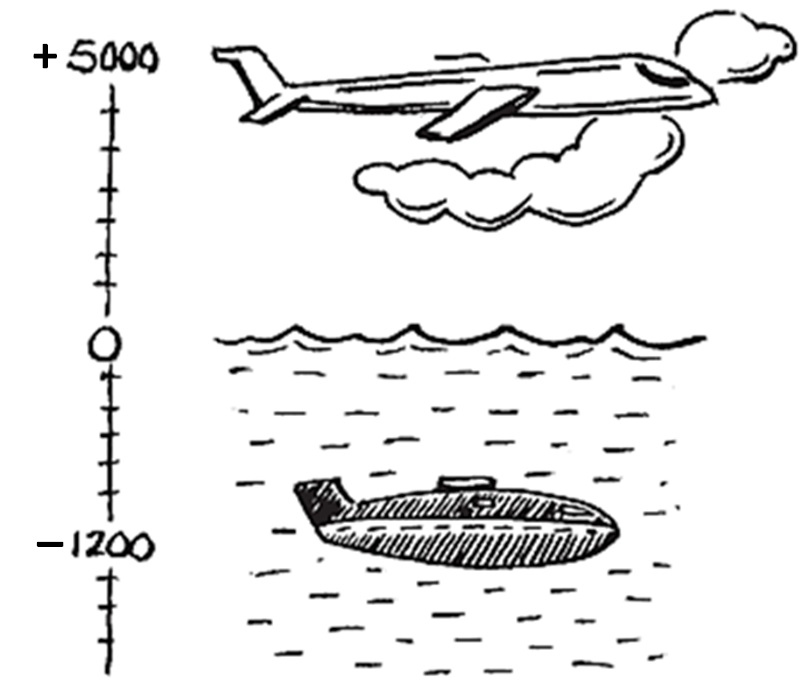Definitions

Chapter 6 Class 6 Integers
Concept wise

Suppose my weight 1 year ago was 95 kg

My weight now is 100 kg

So, I have increased 5 kg

But

If my weight becomes 85 kg

Then, I have reduced 10 kg

But these 5 kg & 10 kg aren’t the same

One is positive, + 5 kg

Other is negative , −10 kg

Similarly

If we deposit 200 rupees to the bank

It is positive, +200

If we withdraw 500 rupees from the bank,

It is negative −500

SimilarlyPlane is 5000 m above sea level .

It is positive, + 5000 m

Submarine is 1200 m below sea level .

It is negative , −1 2 00 m.

Therefore ,

Just like positive numbers : 1, 2, 3, 4, 5, …...

We have negative numbers : −1, −2, −3, −4, −5, …….